Next: Group Velocity Up: Galactic Scale Star Formation Previous: Spiral Structure   Contents

# Density Wave TheoryWe have derived the dispersion relation of the gravitational instability in the rotating thin disk as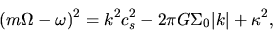(3.36)

where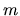represents the number of spiral arms. Although the stability of the stellar system is a little different, we assume this is valid for the stellar system after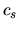is replaced to the velocity dispersion. Since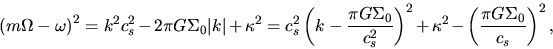(3.37)

we obtain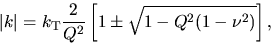(3.38)

where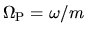is a pattern speed,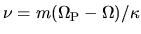is the normalized frequency,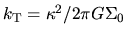is the Toomre's critical wavenumber for a cold (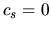) system.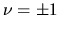, which leads to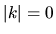, represents the Lindbrad resonance and is rewriten as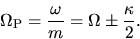(3.39)

Assuming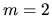, the resonance when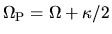is called outer Lindbrad resonance while that of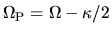is called inner Lindbrad resonance.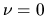means the co-rotation resonance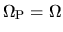. Plotting the wavenumber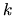against the normalized frequency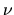of equation (3.38) as Figure 3.7(left), it is shown that, in the case of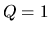, the wavenumber exists for all. Since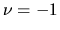, 0, and +1 correspond to the points of ILR, CR, and OLR and these three resonance points appear in accordance with the radial distance, the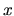-axis of Figure 3.7(left) seems to correspond to the radial distance from the center. In the case of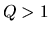, it is shown that a forbidden region appears around the co-rotation resonance point. Waves cannot propagate into the region. Figure 3.7(left) shows that the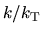has two possible wavenumbers in the permitted region. The waves with largerand smallerare called short waves and long waves, respectively. Consider a wave expressed by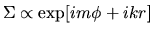. If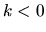, moving from a point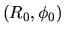in the direction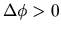and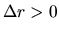the phase difference between the two points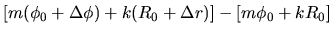can be equal to zero. That is, in the case ofthe wave is leading. On the other hand, if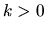, moving in the direction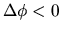andthe phase will be unchanged. In this case, the wave pattern is trailing. Since the dispersion relation is symmetric forand, there are two waves, trailing waves and leading waves. Therefore there are four waves: a short trailing wave, a long trailing wave, a short leading wave, and a long leading wave.

SubsectionsNext: Group Velocity Up: Galactic Scale Star Formation Previous: Spiral Structure   Contents
Kohji Tomisaka 2007-07-08